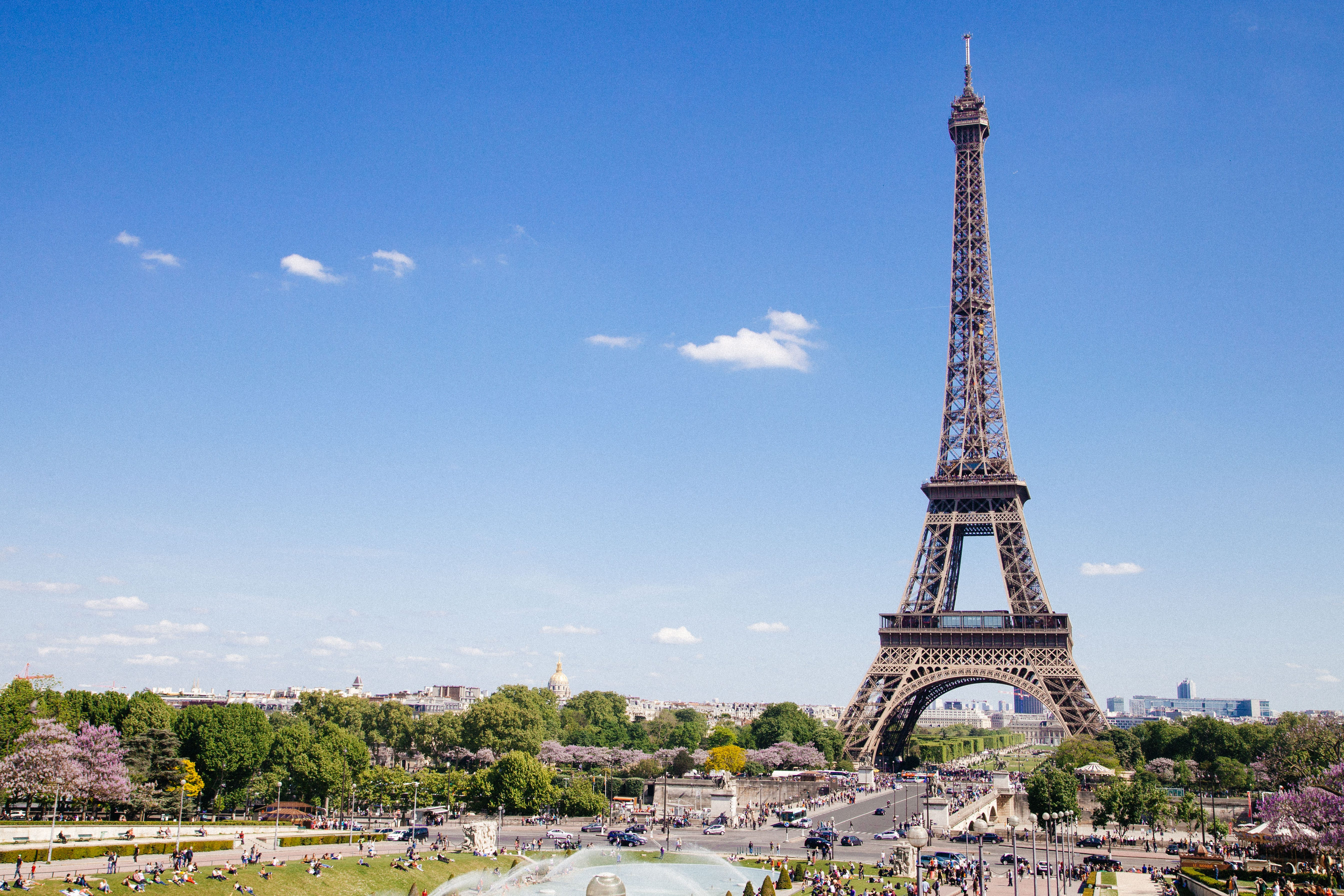# Segmentation and Clustering of neighborhoodsPhoto by Anthony DELANOIX on Unsplash

# 2. Data wrangling

`french_df.shape`

# 3. Methodology

`french_df.dropna(axis =0,inplace = True)french_df.shape`

# 4.Result

`def optimalK(data,max_cluster):        result_df = pd.DataFrame({'gap':[],'cluster_count':[]})    n_ref = 500    gap_arr = np.zeros(max_cluster-1)        original_inertia = np.zeros(max_cluster-1)    reference_inertia = np.zeros(max_cluster-1)        for gap_index,k in enumerate(range(1,max_cluster)):        ref_set = np.zeros(n_ref)                for i in range(n_ref):            ref_dist = np.random.random_sample(data.shape)                        km  = KMeans(k)            km.fit(ref_dist)                        ref_inertia = km.inertia_            ref_set[i] = ref_inertia                    km_orig = KMeans(k)        km_orig.fit(data)                orig_inertia = km.inertia_        original_inertia[gap_index] = np.log(orig_inertia)        reference_inertia[gap_index] = np.mean([np.log(x) for x in ref_set])                # calculate  gap statistics        gap_statistics = np.mean([np.log(x) for x in ref_set]) - np.log(orig_inertia)        gap_arr[gap_index] = gap_statistics                result_df = result_df.append({'gap':gap_statistics,'cluster_count':k},ignore_index=True)            return original_inertia,reference_inertia,gap_arr.argmax()+1,result_df`
`ax = plt.figure(figsize = (8,6))plt.plot(gap_df.cluster_count,gap_df.gap,linewidth = 3)plt.scatter(gap_df[gap_df.cluster_count == optimal_k].cluster_count,gap_df[gap_df.cluster_count == optimal_k].gap,s = 250,c ='r')plt.grid()plt.show()`

Final year student at IIITS | ML | Data Science | Deep Learning

## More from Chandan Roy

Final year student at IIITS | ML | Data Science | Deep Learning